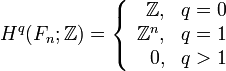# Group cohomology of free groups

View group cohomology of group families | View other specific information about free group

We discuss here the group homology and cohomology for the free group$F_n$ on a freely generating set of size$n$.

## Homology groups for trivial group action

FACTS TO CHECK AGAINST (homology group for trivial group action):
First homology group: first homology group for trivial group action equals tensor product with abelianization
Second homology group: formula for second homology group for trivial group action in terms of Schur multiplier and abelianization|Hopf's formula for Schur multiplier
General: universal coefficients theorem for group homology|homology group for trivial group action commutes with direct product in second coordinate|Kunneth formula for group homology

### Over the integers$H_q(F_n;\mathbb{Z}) = \left\lbrace \begin{array}{rl} \mathbb{Z}, & q = 0 \\ \mathbb{Z}^n, & q = 1 \\ 0, & q > 1 \\\end{array}\right.$

### Over an abelian group

The homology groups over an abelian group$M$ for the trivial group action are as follows:$H_q(F_n;M) = \left\lbrace \begin{array}{rl} M, & q = 0 \\ M^n, & q = 1 \\ 0, & q > 1 \\\end{array}\right.$

## Homology groups for trivial group action

FACTS TO CHECK AGAINST (cohomology group for trivial group action):
First cohomology group: first cohomology group for trivial group action is naturally isomorphic to group of homomorphisms
Second cohomology group: formula for second cohomology group for trivial group action in terms of Schur multiplier and abelianization
In general: dual universal coefficients theorem for group cohomology relating cohomology with arbitrary coefficientsto homology with coefficients in the integers. |Cohomology group for trivial group action commutes with direct product in second coordinate | Kunneth formula for group cohomology

### Over the integers$H^q(F_n;\mathbb{Z}) = \left\lbrace \begin{array}{rl} \mathbb{Z}, & q = 0 \\ \mathbb{Z}^n, & q = 1 \\ 0, & q > 1 \\\end{array}\right.$

### Over an abelian group

The cohomology groups over an abelian group$M$ for the trivial group action are as follows:$H^q(F_n;M) = \left\lbrace \begin{array}{rl} M, & q = 0 \\ M^n, & q = 1 \\ 0, & q > 1 \\\end{array}\right.$